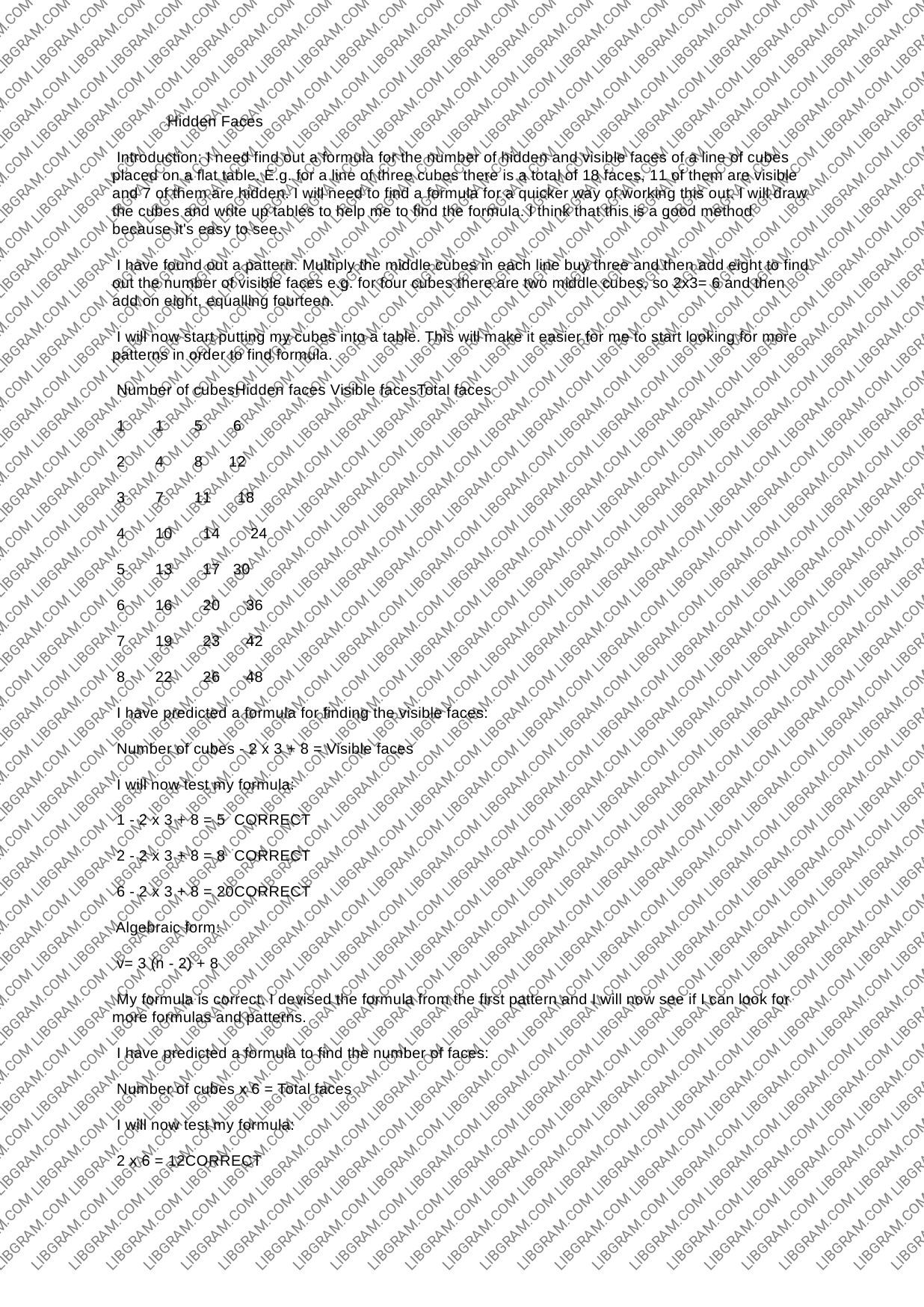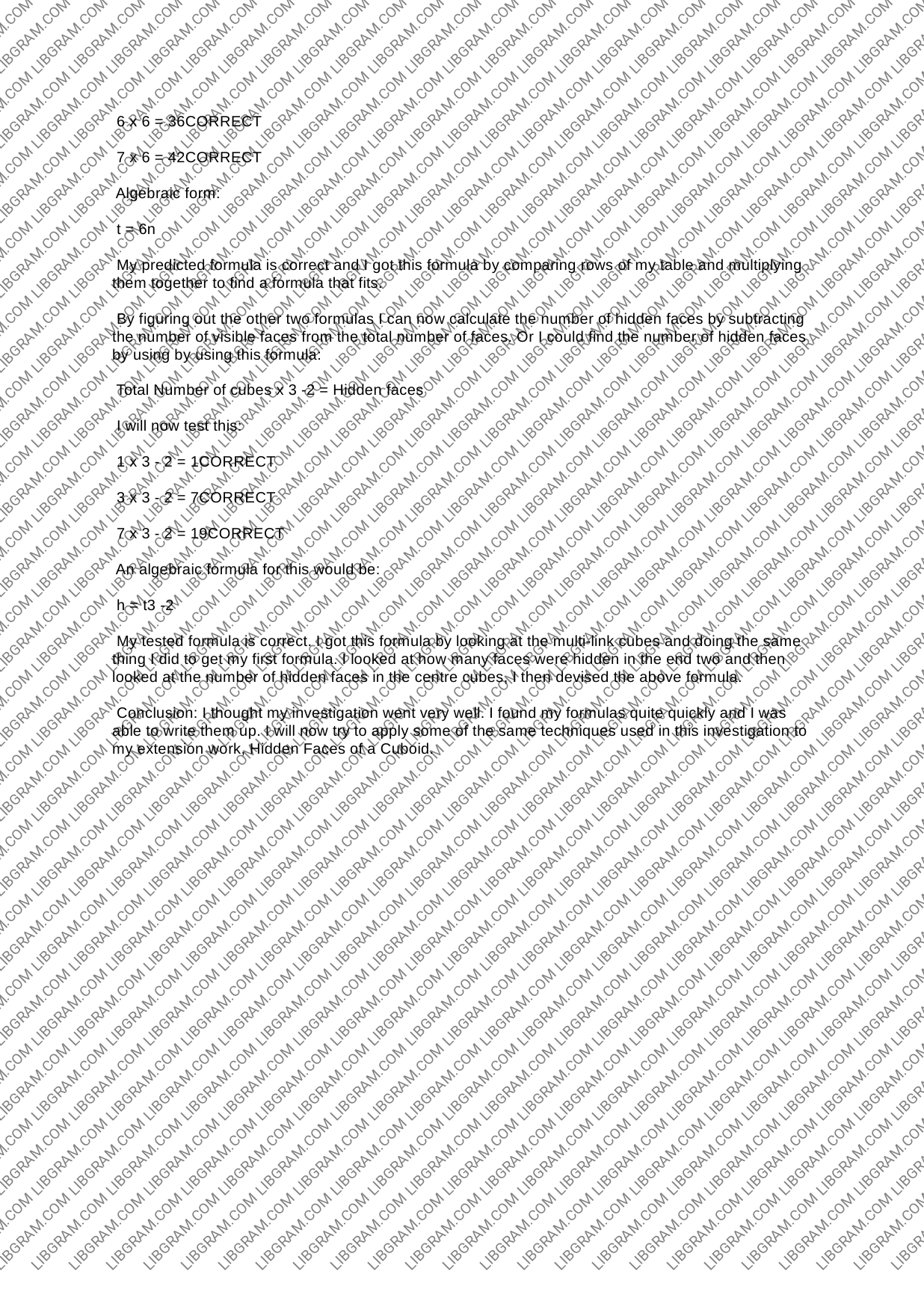# Paper: How to Write Hidden Faces of the CuboidsHidden Faces Introduction: | need find out a formula for the number of hidden and visible faces of a line of cubes placed on a flat table. E.g. for a line of three cubes there is a total of 18 faces, 11 of them are visible and 7 of them are hidden. | will need to find a formula for a quicker way of working this out. | will draw the cubes and write up tables to help me to find the formula. | think that this is a good method because it's easy to see. | have found out a pattern. Multiply the middle cubes in each line buy three and then add eight to find out the number of visible faces e.g. for four cubes there are two middle cubes, so 2x3= 6 and then add on eight, equalling fourteen. | will now start putting my cubes into a table. This will make it easier for me to start looking for more patterns in order to find formula. Number of cubesHidden faces Visible facesTotal faces 1 1 5 6 2 4 8 12 3 7 i. 18 4 10 14 24 5 13 17 30 6 16 20 36 7 19 23° «42 8 22 26 48 | have predicted a formula for finding the visible faces: Number of cubes - 2 x 3 + 8 = Visible faces | will now test my formula: 1-2x3+8=5 CORRECT 2-2x3+8=8 CORRECT 6-2x3+8=20CORRECT Algebraic form: v=3(n-2)+8 My formula is correct. | devised the formula from the first pattern and | will now see if | can look for more formulas and patterns. | have predicted a formula to find the number of faces: Number of cubes x 6 = Total faces | will now test my formula: 2x 6 = 12CORRECT 6 x 6 = 36CORRECT 7x 6 = 42CORRECT Algebraic form: t=6n My predicted formula is correct and | got this formula by comparing rows of my table and multiplying them together to find a formula that fits. By figuring out the other two formulas | can now calculate the number of hidden faces by subtracting the number of visible faces from the total number of faces. Or | could find the number of hidden faces by using by using this formula: Total Number of cubes x 3 -2 = Hidden faces | will now test this: 1x3-2=1CORRECT 3x 3-2=7CORRECT 7x3-2=19CORRECT An algebraic formula for this would be: h=t3-2 My tested formula is correct. | got this formula by looking at the multi-link cubes and doing the same thing | did to get my first formula. | looked at how many faces were hidden in the end two and then looked at the number of hidden faces in the centre cubes, | then devised the above formula. Conclusion: | thought my investigation went very well. | found my formulas quite quickly and | was. able to write them up. | will now try to apply some of the same techniques used in this investigation to my extension work, Hidden Faces of a Cuboid. ...